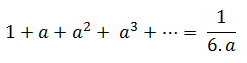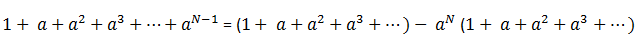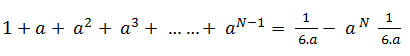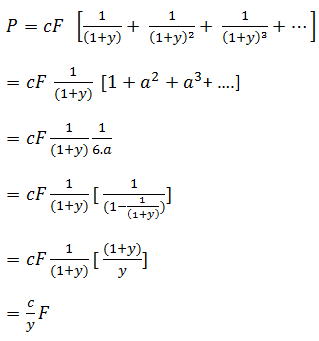# Infinite Series and Its Applications

Bonds consist of fixed coupons and the pricing of the bond involves the use of combinations of infinite series.  A typical example would be the sum of terms that increase at an infinite rate.This formula can be proved by multiplying both sides of the equation by (1-a) and then cancelling common terms on both sides.

A geometric series with a finite number of terms N can be written as the difference between two infinite series such that all the terms with order N or higher will cancel each other.The above equation can then be rewritten as follows:The above formulas can be used to value bonds. The derivation of the formula for consols with fixed coupon rate c, yield y and face value F is given below. A consol is an instrument which has an infinite number of coupon payments at the specified coupon rate –